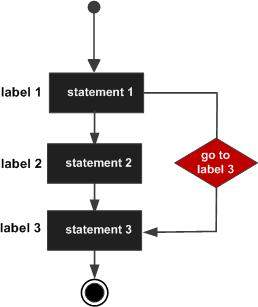# C++ goto statement

A goto statement provides an unconditional jump from the goto to a labeled statement in the same function.

NOTE − Use of goto statement is highly discouraged because it makes difficult to trace the control flow of a program, making the program hard to understand and hard to modify. Any program that uses a goto can be rewritten so that it doesn't need the goto.

## Syntax

The syntax of a goto statement in C++ is −

```goto label;
..
.
label: statement;
```

Where label is an identifier that identifies a labeled statement. A labeled statement is any statement that is preceded by an identifier followed by a colon (:).

## Flow Diagram## Example

```#include <iostream>
using namespace std;

int main () {
// Local variable declaration:
int a = 10;

// do loop execution
LOOP:do {
if( a == 15) {
// skip the iteration.
a = a + 1;
goto LOOP;
}
cout << "value of a: " << a << endl;
a = a + 1;
}
while( a < 20 );

return 0;
}
```

When the above code is compiled and executed, it produces the following result −

```value of a: 10
value of a: 11
value of a: 12
value of a: 13
value of a: 14
value of a: 16
value of a: 17
value of a: 18
value of a: 19
```

One good use of goto is to exit from a deeply nested routine. For example, consider the following code fragment −

```for(...) {
for(...) {
while(...) {
if(...) goto stop;
.
.
.
}
}
}
stop:
cout << "Error in program.\n";
```

Eliminating the goto would force a number of additional tests to be performed. A simple break statement would not work here, because it would only cause the program to exit from the innermost loop.

cpp_loop_types.htm